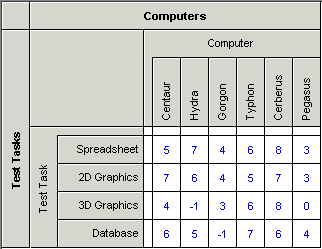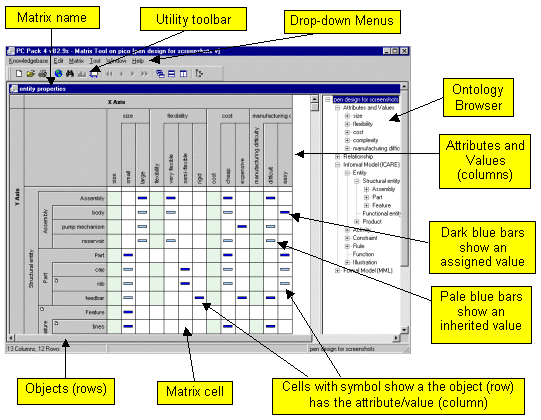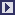## PCPACK6 Matrix Tool

 The Matrix tool allows two types of matrix (aka grid) to be created and edited: an Attribute Matrix and a Relationship Matrix. Basic Features Attribute Matrix or Relationship Matrix Simple clicking operation for assigning properties (in an Attribute Matrix) or asserting relationships (in a Relationship Matrix) Inheritance of attributes/values (in an Attribute Matrix), and indication of conflicts (if multiple inheritance is present) Selectable displays for relationship attributes  Desigable 'user forms' for data entry to a Relationship Matrix Customisable symbols for numerical relationship values in a Relationship Matrix  Charts for displaying numerical values of a Relationship Matrix Attribute Matrix An Attribute Matrix is a way of associating properties (attributes and values) to knowledge objects. It does this by presenting a matrix of knowledge objects on the vertical axis and attributes/values on the horizontal axis. A simple clicking operation associates attributes and/or values with the relevant object. An example of an Attribute Matrix is shown below.Relationship Matrix A Relationship Matrix is a way of associating certain knowledge objects (on the X-axis) to certain knowledge objects (on the Y-axis) using a specified relation, such as "followed by", "has constraint" or "requires". One relation per matrix is used. If the relation chosen has properties (attributes and values) then special symbols can be used to show each relationship’s properties. User forms can be designed to aid entry of data, and charts can be created to display the numerical properties of relationships in graphical form. An example of a Relationship Matrix is shown below.Another example of a Relationship Matrix is shown below. This matrix shows the numerical values of a relation attribute.Another example of a Relationship Matrix is shown below. This matrix shows symbols for the ranges of numerical values for a relation attribute.User Interface The user interface of the Matrix Tool is explained in the following figure.Other PCPACK tools:Protocol ToolLadder ToolDiagram ToolAnnotation ToolAdmin ToolPublisher ToolDiagram Template ToolEquation Editor ToolLauncher Wizard Tool